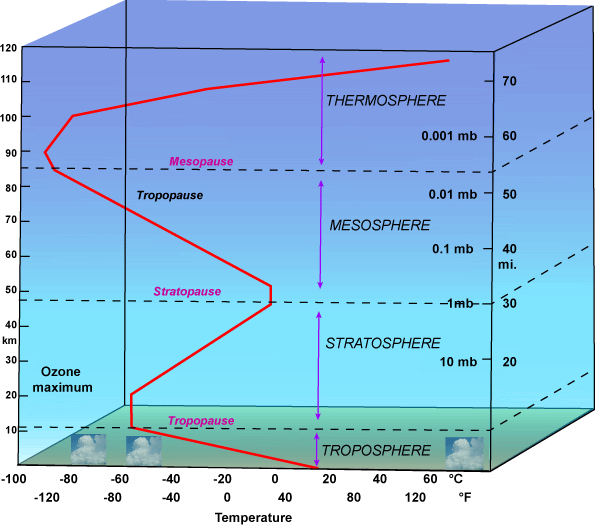## Friday, May 01, 2015

### Heat Changes Due to UAH's New DatasetSo how much less heat is in the lower troposphere (than what we thought) because of the changes in UAH's new dataset?

Very little.

The trend of the new dataset, from its beginning in Dec 1978 to March 2015 is +0.114 C/decade. The trend of their previous dataset was +0.140 C/decade.

Since the dataset is 36.33 years long, the new dataset has about 0.41°C of warming, and the old dataset had 0.51°C. So the difference is 0.1°C.

To get a sense of how much change in heat this represents, I'll assume the mass of the lower troposphere is the mass of the entire atmosphere (since it gets exponentially thinner as you go up in height), which is 5e18 kg. (It's easily estimated from surface pressure: g*matmo = Ps*surface_area_of_Earth, where g is the acceleration due to gravity at the surface.)

The heat capacity of air decreases below Earth's surface temperature, but not by alot, so I'll take it to be the average over the range, 1.0 kJ/kgK.

The atmosphere is 0.25% water by weight, and water vapor has a specific heat of less than 2 times dry air, so I'm going to ignore its presence in the atmosphere. (This is an order of magnitude calculation only.)

Then the heat change is ΔQ = mcΔT ~ 5e20 J, or, over the 36.3 years, ~ -4e11 Watts. Dividing by the Earth's surface, that comes to ~ -0.001 W/m2.

That's about the same as comes from the Earth's interior, which is 86 mW/m2.

So in terms of a planetary energy imbalance, this isn't a significant change at all -- it won't have been noticeable through changes in ocean heat content, which cannot be used to notice a missing milliwatt per square meter. (Added 5/2: And only about 4 times this much heat total has gone into the lower troposphere in the last 36+ years -- less than 0.01 W/m2.)

Of course, though the ocean is the best diagnostic for a planetary energy imbalance ("Global warming is ocean warming," says NOAA oceanographer Greg Johnson), we don't live in the ocean. But we also don't live atop it, and for the planet's land (well, the lower troposphere above it) UAH's new dataset has a trend of +0.19 C/decade.

And it's +0.20 C/decade for the Northern Hemisphere land. But the "2°C limit" refers to the globe, not just land.